Question

# An object is located 15.2 cm in front of a convex mirror, the image being 7.79...

An object is located 15.2 cm in front of a convex mirror, the image being 7.79 cm behind the mirror. A second object, twice as tall as the first one, is placed in front of the mirror, but at a different location. The image of this second object has the same height as the other image. How far in front of the mirror is the second object located?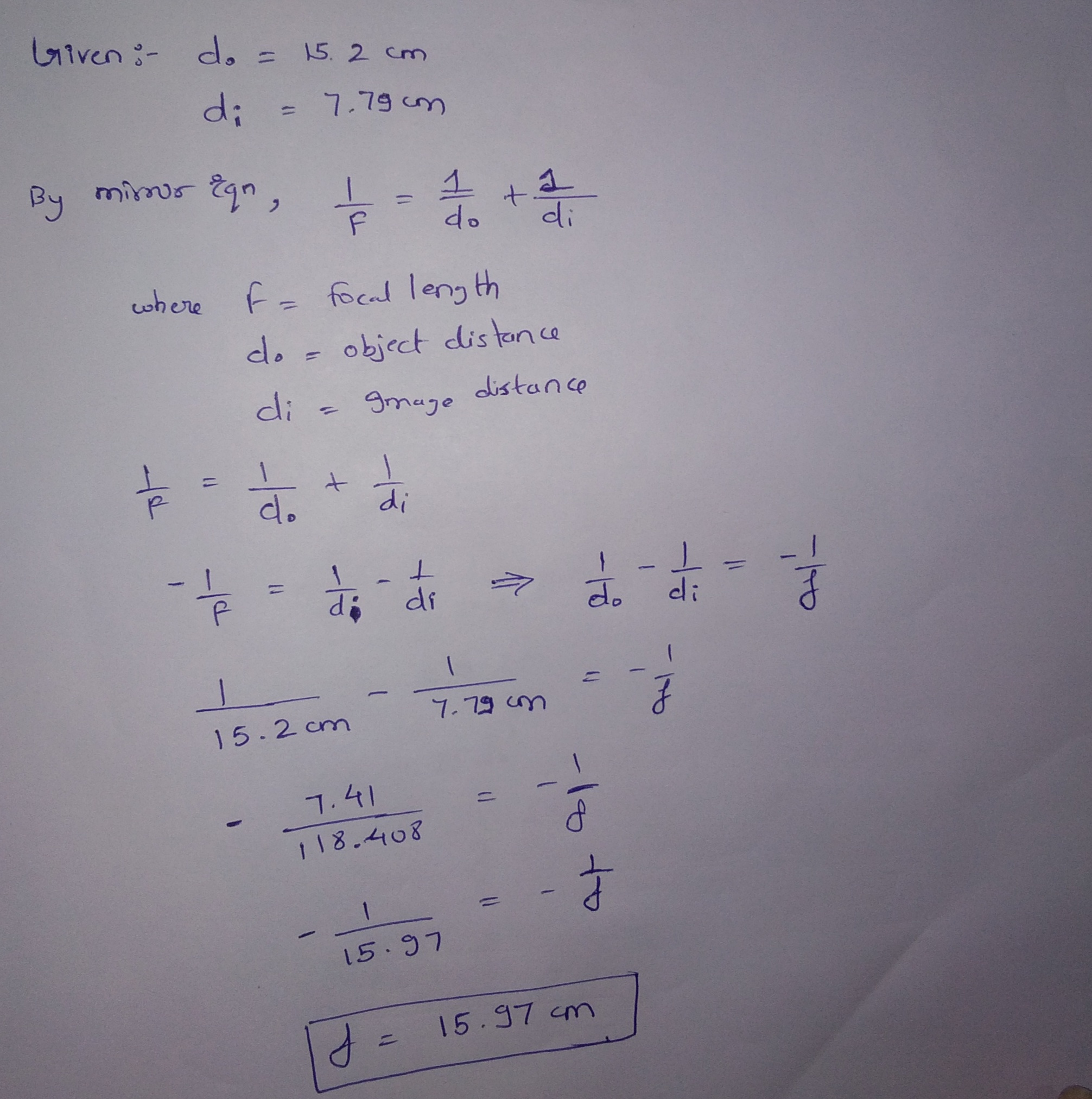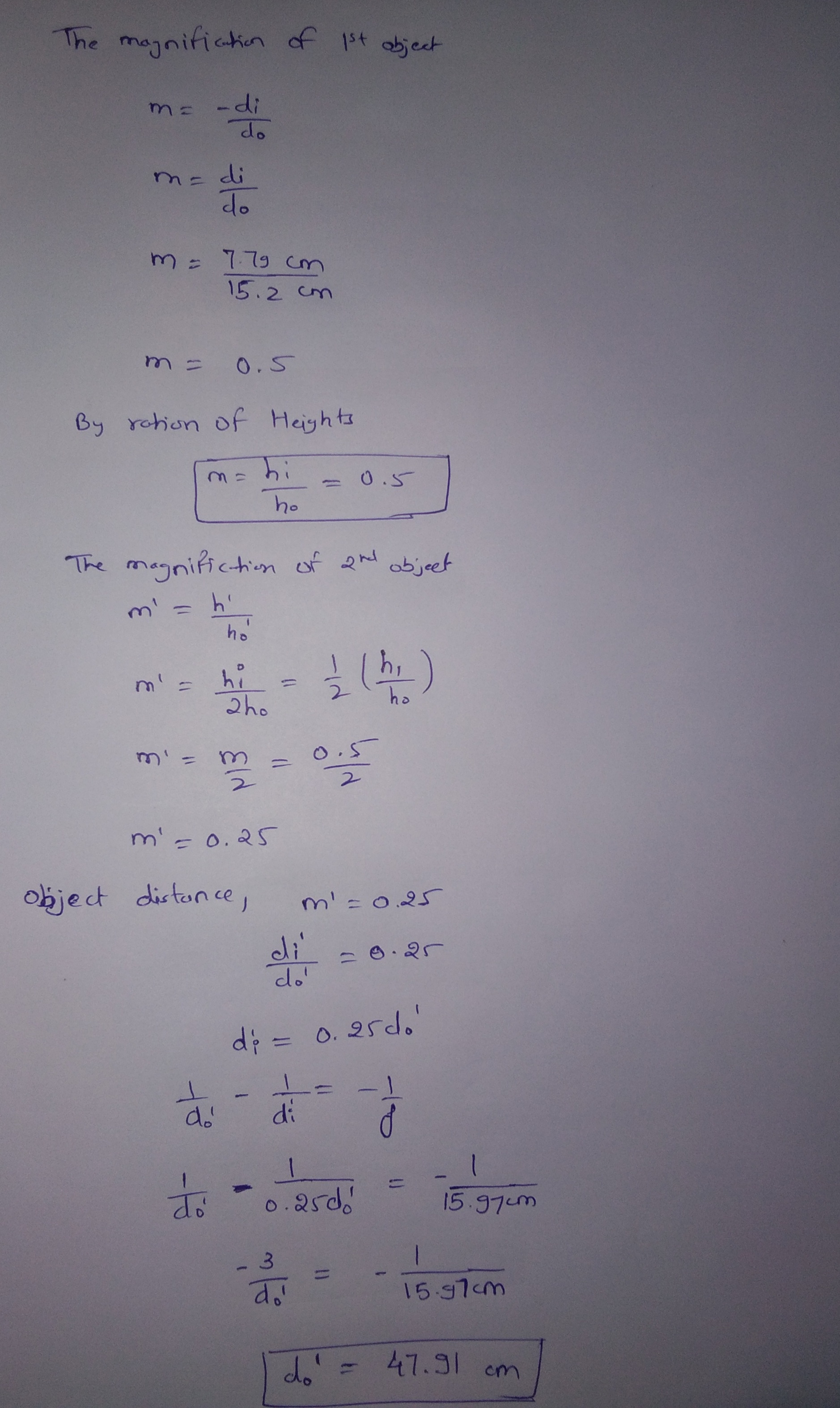#### Earn Coins

Coins can be redeemed for fabulous gifts.

Similar Homework Help Questions
• ### 7. An object is located 14.5 cm in front of a convex mirror, the image being...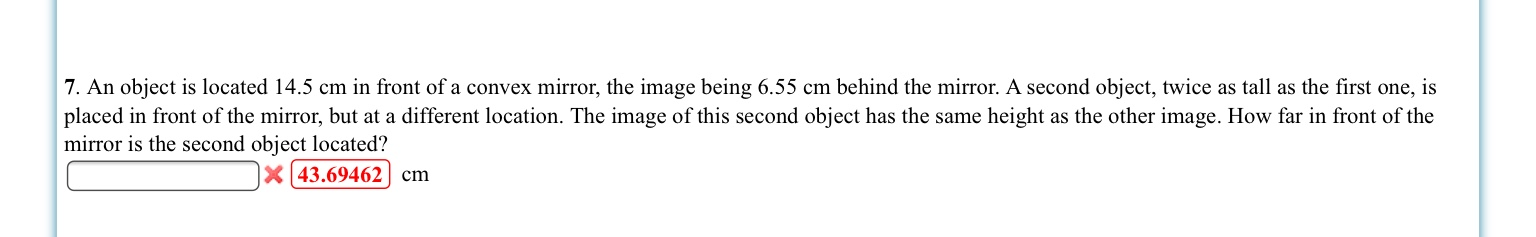7. An object is located 14.5 cm in front of a convex mirror, the image being 6.55 cm behind the mirror. A second object, twice as tall as the first one, is placed in front of the mirror, but at a different location. The image of this second object has the same height as the other image. How far in front of the mirror is the second object located? X43.69462 cm

• ### An object that is 28 cm in front of a convex mirror has an image located...

An object that is 28 cm in front of a convex mirror has an image located 16 cm behind the mirror. How far behind the mirror is the image located when the object is 19 cm in front of the mirror?

• ### An object that is 34.0 cm in front of a convex mirror has an image located...

An object that is 34.0 cm in front of a convex mirror has an image located 17.0 cm behind the mirror. What is the distance of the image when the object is 36.0 cm in front of the mirror? cm

• ### an object that is 25cm in front of a convex mirror has an image located 17cm...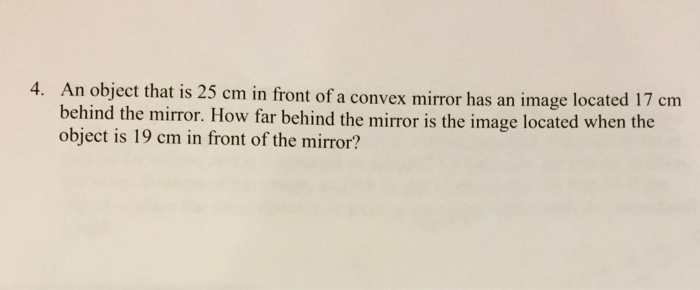an object that is 25cm in front of a convex mirror has an image located 17cm behind the mirror. how far behind the mirror is the image located when the object is 19cm in front of the mirror? Please show all work, and explanation. My professor doesn't explain this well at all. Thank you!

• ### 1. The image of an object is 11.1 cm behind a convex mirror when the object...

1. The image of an object is 11.1 cm behind a convex mirror when the object is far from the mirror. Determine the absolute value of the distance from the mirror to the image when the object is placed 2.5 cm in front of the mirror. ______ cm 2. If when an object is placed 13.5 cm in front of a mirror the image is located 21.7 cm behind the mirror, determine the following. The focal length of the mirror....

• ### When an object is located very far away from a convex mirror, the image of the...

When an object is located very far away from a convex mirror, the image of the object is 12.0 cm behind the mirror. Using a ray diagram drawn to scale, determine where the image is located when the object is placed 3.0 cm in front of the mirror. Note that the mirror must be drawn to scale also. In your drawing, assume that the height of the object is 3.0 cm.

• ### When an object is located very far away from a convex mirror, the image of the...

When an object is located very far away from a convex mirror, the image of the object is 20.0 cm behind the mirror. Using a ray diagram drawn to scale, determine where the image is located when the object is placed 6.7 cm in front of the mirror. Note that the mirror must be drawn to scale also. In your drawing, assume that the height of the object is 3.0 cm. first I got 2.25 and 4.01. both answers are...

• ### 42. I A 1.0-cm-tall object is 20 cm in front of a convex mirror that has...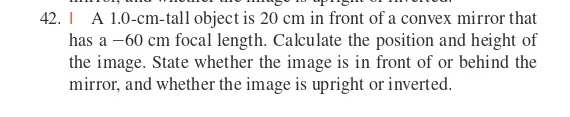42. I A 1.0-cm-tall object is 20 cm in front of a convex mirror that has a -60 cm focal length. Calculate the position and height of the image. State whether the image is in front of or behind the mirror, and whether the image is upright or inverted.

• ### A convex mirror has a radius of curvature of 45.0 cm. A 10.7-cm-tall object is placed...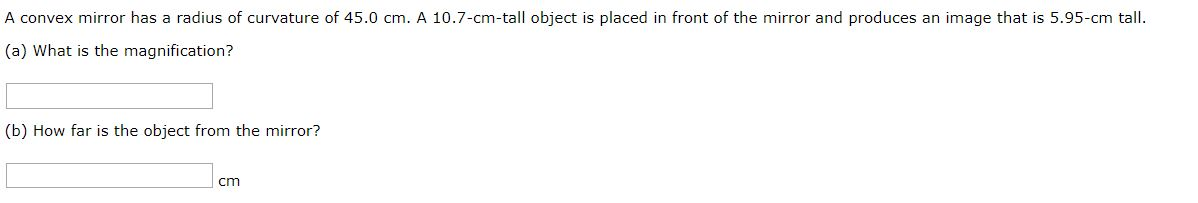A convex mirror has a radius of curvature of 45.0 cm. A 10.7-cm-tall object is placed in front of the mirror and produces an image that is 5.95-cm tall. (a) What is the magnification? (b) How far is the object from the mirror? cm

• ### A 5 cm tall object is placed 8 cm in front of a convex mirror of...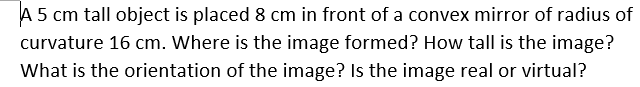A 5 cm tall object is placed 8 cm in front of a convex mirror of radius of curvature 16 cm. Where is the image formed? How tall is the image? What is the orientation of the image? Is the image real or virtual?